Next: 13.3 Cones
Up: 13 Polyhedra
Previous: 13.1 Regular Polyhedra

# 13.2 Cylinders

Given a line L and a curve C in a plane P, the cylinder with generator L and directrix C is the surface obtained by moving L parallel to itself, so that a point of L is always on C. If L is parallel to the z-axis, the surface's implicit equation does not involve the variable z. Conversely, any implicit equation that does not involve one of the variables (or that can be brought to that form by a change of coordinates) represents a cylinder.

If C is a simple closed curve, we also apply the word cylinder to the solid enclosed by the surface generated in this way (Figure 1, left).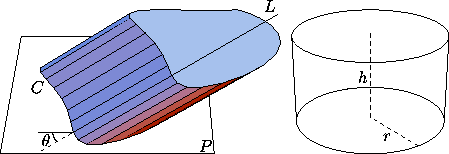Figure 1: Left: an oblique cylinder with generator L and directrix C. Right: a right circular cylinder.

The volume contained between P and a plane P' parallel to P is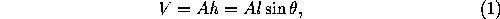where A is the area in the plane P enclosed by C, h is the distance between P and P' (measured perpendicularly), l is the length of the segment of L contained between P and P', and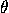is the angle that L makes with P. When=90° we have a right cylinder, and h=l. For a right cylinder, the lateral area between P and P' is hs, where s is the length (circumference) of C.

The most important particular case is the right circular cylinder (often simply called a cylinder). If r is the radius of the base and h is the altitude (Figure 1, right), the lateral area is

2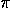rh,

the total area is 2r(r+h), and the volume isr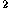h.

The implicit equation of this surface can be written

x+y=r;

Next: 13.3 Cones
Up: 13 Polyhedra
Previous: 13.1 Regular PolyhedraThe Geometry Center Home Page

Silvio Levy
Wed Oct 4 16:41:25 PDT 1995

This document is excerpted from the 30th Edition of the CRC Standard Mathematical Tables and Formulas (CRC Press). Unauthorized duplication is forbidden.